# Mining on Manifolds: Metric Learning without Labels

This paper  proposes an unsupervised framework for hard training-example mining. The proposed framework has two phases. Given a collection of unlabelled images, the first phase identifies positive and negative image pairs. Then, the second phase leverages these pairs to fine-tune a pretrained network.

Phase #1:

The first phase leverage a pretrained network to project the unlabelled images into an embedding space (Manifold) as shown in Fig.1.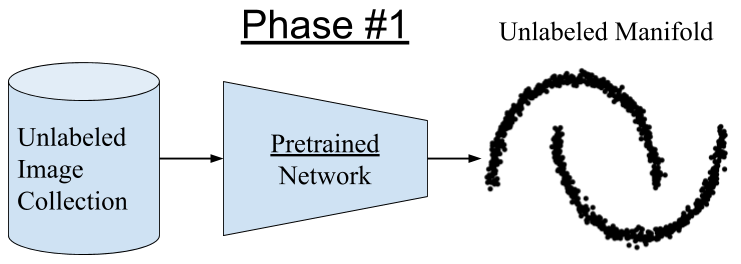Figure 1: A pretrained network embeds images into a manifold (feature space)

The manifold is used to create pairs/triplets from the unlabeled images. For an anchor image, the manifold provides two types of nearest neighbors: Euclidean (NN^e) and Manifold (NN^m) as shown in Fig.2. The figure highlights a single anchor image in black. The NN^e are highlighted in orange, while the NN^m are highlighted in purple. For an anchor image, hard negatives are identified by removing the NN^m from the NN^e. Similarly, the hard positives are identified by removing the NN^e from the NN^m.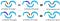Figure 2: Identify hard positive and negative images using the Euclidean and manifold nearest neighbors.

At the end of phase #1, every anchor image will have a list of hard negatives and another list for hard positives. These negatives and positives are used to create pairs/triplets for training a new network in a supervised manner.

It is worth noting that the anchor images are not selected randomly. Instead, the paper picks anchors that (1) are diverse, and (2) have many relevant images in the collection. These anchors are identified by computing the *stationary probability distribution* of a random walk on the unlabeled manifold. The probability reflects the importance of each node in the graph, as expressed by the probability of a random walker visiting it.

Phase #2:

Once the pairs/triplets are available, it is straight forward to train a new deep network using contrastive or triplet loss. These are metric-learning losses that learn a feature embedding where objects from the same class are closer than objects from different classes. Accordingly, retrieval is used to evaluate the proposed method. For example, the proposed method is evaluated using the CUB-200 (Birds) dataset. The proposed method does not use the image labels in this dataset. Yet, the method delivers a competitive performance compared to supervised methods as shown in Table.1.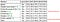Table 1: Recall@k and NMI on CUB-200–2011. All methods except for Ours and cyclic match  use ground-truth labels during training.

The triplets identified by phase #1 are qualitatively evaluated against the Euclidean nearest neighbor (NN^e) baseline. Fig.3 compares the positive and negative triplets identified by the proposed framework.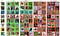Figure 3: Sample CUB-200-2011 anchor images (x^r), positive images from the proposed method (P^+(x^r)) and baseline (NN^e_3(x^r)), and negative images from the proposed method (P^−(x^r)) and baseline (X \ NN^e_3(x^r)). The baseline is Euclidean nearest neighbors and non-neighbors. Positive (negative) ground-truth framed in green (red). Labels are only used for qualitative evaluation and not during training. NN^e_3(x^r) indicates the three euclidean nearest neighbors to the anchor image x^r.

The paper presents more quantitative and qualitative evaluations using other datasets. However, the core idea remains the same. It is possible to use a pretrained network to create pairs/triplets for unlabeled image collection. These pairs/triplets are then used to train a deep network in a supervised manner.

1. [Strength] The paper is well written and tackles an interesting problem — training a network on unlabeled image collection. The authors released their implementation on Github.
2. [Strength] The paper proposed a specific approach to identify useful anchor images, i.e., the stationary probability distribution (A). The authors provide an ablation study to evaluate this approach against randomly sampled anchors. The stationary probability distribution (A) approach significantly outperforms random sampling as shown in the next Table.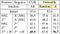Impact of choices of anchors and pools of positive and negative examples on Recall@1 on CUB-200–2011 and mAP on Oxford5k. Higher Recall/mAP is better. On CUB, all images are used as anchors, while on Oxford5K anchors are selected either at random or by the stationary probability distribution (A) approach. The positive and negative pools are formed by either the baseline with Euclidean nearest neighbors (NN^e) or the proposed hard-mining selection framework (P+ and P−).

3. [Weakness] The paper uses the word *unsupervised* to describe their framework. Technically, their framework is not unsupervised because the framework leverages a pretrained network. At phase #1, a pretrained network is vital to generate the unlabeled manifold. This pretrained network is trained using labeled images.

4. To find the positive and negative pairs, the unlabeled manifold is transformed into a graph with nodes and edges. This graph delivers the manifold nearest neighbors (NN^m). Processing this graph increases the computational cost. According to the paper, it takes 80min on 12 CPU threads for a 1M retrieval training set. It would be interesting to eliminate or reduce this computational cost.

Resources

 Ahmet Iscen, Giorgos Tolias, Yannis Avrithis, Ondrej Chum. Mining on Manifolds: Metric Learning without Labels. CVPR 2018

I write reviews on computer vision papers. Writing tips are welcomed.

## More from Ahmed Taha

I write reviews on computer vision papers. Writing tips are welcomed.